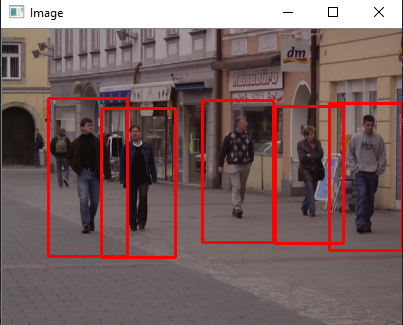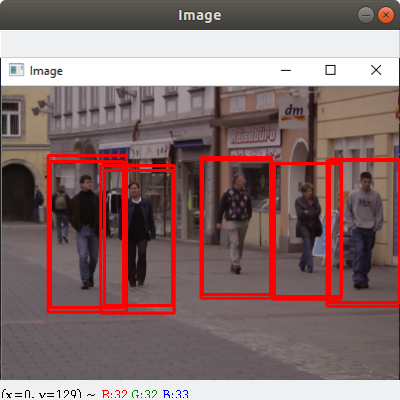# Pedestrian Detection using OpenCV-Python

OpenCV is an open-source library, which is aimed at real-time computer vision. This library is developed by Intel and is cross-platform – it can support Python, C++, Java, etc. Computer Vision is a cutting edge field of Computer Science that aims to enable computers to understand what is being seen in an image. OpenCV is one of the most widely used libraries for Computer Vision tasks like face recognition, motion detection, object detection, etc. In this tutorial, we are going to build a basic Pedestrian Detector for images and videos using OpenCV. Pedestrian detection is a very important area of research because it can enhance the functionality of a pedestrian protection system in Self Driving Cars. We can extract features like head, two arms, two legs, etc, from an image of a human body and pass them to train a machine learning model. After training, the model can be used to detect and track humans in images and video streams. However, OpenCV has a built-in method to detect pedestrians. It has a pre-trained HOG(Histogram of Oriented Gradients) + Linear SVM model to detect pedestrians in images and video streams.

This algorithm checks directly surrounding pixels of every single pixel. The goal is to check how darker is the current pixel compared to the surrounding pixels. The algorithm draws and arrows showing the direction of the image getting darker. It repeats the process for each and every pixel in the image. At last, every pixel would be replaced by an arrow, these arrows are called Gradients. These gradients show the flow of light from light to dark. By using these gradients algorithms perform further analysis. To learn more about HOG, read Navneet Dalal and Bill Triggs research paper on HOG for Human Detection..

#### Requirements

```opencv-python 3.4.2

imutils 0.5.3```

To install the above modules type the below command in the terminal.

```pip install opencv-python 3.4.2
pip install imutils 0.5.3```

Example 1:

Lets make the program to detect pedestrians in an Image:

Image Used:## Python3

 `import` `cv2` `import` `imutils` ` `  `# Initializing the HOG person` `# detector` `hog ``=` `cv2.HOGDescriptor()` `hog.setSVMDetector(cv2.HOGDescriptor_getDefaultPeopleDetector())` ` `  `# Reading the Image` `image ``=` `cv2.imread(``'img.png'``)` ` `  `# Resizing the Image` `image ``=` `imutils.resize(image,` `                       ``width``=``min``(``400``, image.shape[``1``]))` ` `  `# Detecting all the regions in the ` `# Image that has a pedestrians inside it` `(regions, _) ``=` `hog.detectMultiScale(image, ` `                                    ``winStride``=``(``4``, ``4``),` `                                    ``padding``=``(``4``, ``4``),` `                                    ``scale``=``1.05``)` ` `  `# Drawing the regions in the Image` `for` `(x, y, w, h) ``in` `regions:` `    ``cv2.rectangle(image, (x, y), ` `                  ``(x ``+` `w, y ``+` `h), ` `                  ``(``0``, ``0``, ``255``), ``2``)`   `# Showing the output Image` `cv2.imshow("Image", image)` `cv2.waitKey(``0``)` ` `  `cv2.destroyAllWindows()`

Output:Example 2: Lets make the program to detect pedestrians in a video:

## Python3

 `import` `cv2` `import` `imutils` ` `  `# Initializing the HOG person` `# detector` `hog ``=` `cv2.HOGDescriptor()` `hog.setSVMDetector(cv2.HOGDescriptor_getDefaultPeopleDetector())` ` `  `cap ``=` `cv2.VideoCapture(``'vid.mp4'``)` ` `  `while` `cap.isOpened():` `    ``# Reading the video stream` `    ``ret, image ``=` `cap.read()` `    ``if` `ret:` `        ``image ``=` `imutils.resize(image, ` `                               ``width``=``min``(``400``, image.shape[``1``]))` ` `  `        ``# Detecting all the regions ` `        ``# in the Image that has a ` `        ``# pedestrians inside it` `        ``(regions, _) ``=` `hog.detectMultiScale(image,` `                                            ``winStride``=``(``4``, ``4``),` `                                            ``padding``=``(``4``, ``4``),` `                                            ``scale``=``1.05``)` ` `  `        ``# Drawing the regions in the ` `        ``# Image` `        ``for` `(x, y, w, h) ``in` `regions:` `            ``cv2.rectangle(image, (x, y),` `                          ``(x ``+` `w, y ``+` `h), ` `                          ``(``0``, ``0``, ``255``), ``2``)` ` `  `        ``# Showing the output Image` `        ``cv2.imshow("Image", image)` `        ``if` `cv2.waitKey(``25``) & ``0xFF` `=``=` `ord``(``'q'``):` `            ``break` `    ``else``:` `        ``break`   `cap.release()` `cv2.destroyAllWindows()`

Output:

Whether you're preparing for your first job interview or aiming to upskill in this ever-evolving tech landscape, GeeksforGeeks Courses are your key to success. We provide top-quality content at affordable prices, all geared towards accelerating your growth in a time-bound manner. Join the millions we've already empowered, and we're here to do the same for you. Don't miss out - check it out now!

Previous
Next